• +91 9971497814
• info@interviewmaterial.com

# Chapter 10 Straight lines Ex-10.2 Interview Questions Answers

### Related Subjects

Question 1 : Write the equations for the x and y-axes.

The y-coordinate of every point on the x-axis is 0.
Therefore, the equation of the x-axis is y = 0.
The x-coordinate of every point on the y-axis is 0.
Therefore, the equation of the y-axis is x = 0.

Question 2 : Find the equation of the line which passes through the point (–4, 3) with slope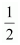.

We know that the equation of the line passing through point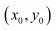, whose slope is m, is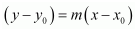.
Thus, the equation of the line passing through point (–4, 3), whose slope is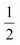, is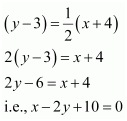Question 3 : Find the equation of the line which passes though (0, 0) with slope m.

We know that the equation of the line passing through point, whose slope is m, is.
Thus, the equation of the line passing through point (0, 0), whose slope is m,is
(y – 0) = m(x – 0)
i.e., y = mx

Question 4 : Find the equation of the line which passes though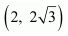and is inclined with the x-axis at an angle of 75°.

The slope of the line that inclines with the x-axis at an angle of 75° is
m = tan 75°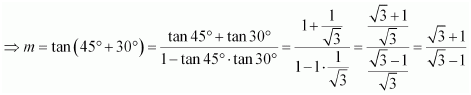We know that the equation of the line passing through point, whose slope is m, is.
Thus, if a line passes thoughand inclines with the x-axis at an angle of 75°, then the equation of the line is given as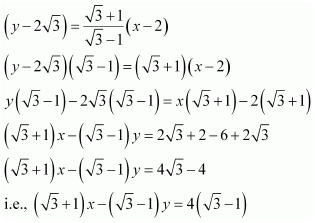Question 5 : Find the equation of the line which intersects the x-axis at a distance of 3 units to the left of origin with slope –2.

It is known that if a line with slope m makes x-intercept d, then the equation of the line is given as
y = m(x – d)
For the line intersecting the x-axis at a distance of 3 units to the left of the origin, d = –3.
The slope of the line is given as m = –2
Thus, the required equation of the given line is
y = –2 [x – (–3)]
y = –2x – 6
i.e., 2x + y + 6 = 0

Question 6 : Find the equation of the line which intersects the y-axis at a distance of 2 units above the origin and makes an angle of 30° with the positive direction of the x-axis.

It is known that if a line with slope m makes y-intercept c, then the equation of the line is given as
y = mx + c
Here, c = 2 and m = tan 30°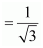.
Thus, the required equation of the given line is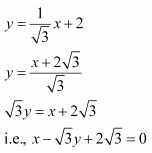Question 7 : Find the equation of the line which passes through the points (–1, 1) and (2, –4).

It is known that the equation of the line passing through points (x1, y1) and (x2, y2) is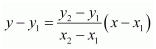.
Therefore, the equation of the line passing through the points (–1, 1) and
(2, –4) is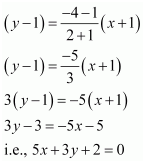Question 8 : Find the equation of the line which is at a perpendicular distance of 5 units from the origin and the angle made by the perpendicular with the positive x-axis is 30°

If p is the length of the normal from the origin to a line and ω is the angle made by the normal with the positive direction of the x-axis, then the equation of the line is given by xcos ω + y sin ω = p.
Here, p = 5 units and ω = 30°
Thus, the required equation of the given line is
x cos 30° + y sin 30° = 5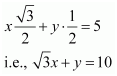Question 9 : The vertices of ΔPQR are P (2, 1), Q (–2, 3) and R (4, 5). Find equation of the median through the vertex R.

It is given that the vertices of ΔPQR are P (2, 1), Q (–2, 3), and R (4, 5).
Let RL be the median through vertex R.
Accordingly, L is the mid-point of PQ.
By mid-point formula, the coordinates of point L are given by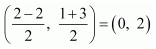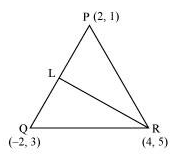It is known that the equation of the line passing through points (x1, y1) and (x2, y2) is.
Therefore, the equation of RL can be determined by substituting (x1, y1) = (4, 5) and (x2, y2) = (0, 2).
Hence,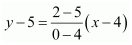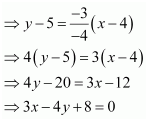Thus, the required equation of the median through vertex R is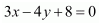.

Question 10 : Find the equation of the line passing through (–3, 5) and perpendicular to the line through the points (2, 5) and (–3, 6).

The slope of the line joining the points (2, 5) and (–3, 6) is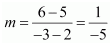We know that two non-vertical lines are perpendicular to each other if and only if their slopes are negative reciprocals of each other.
Therefore, slope of the line perpendicular to the line through the points (2, 5) and (–3, 6)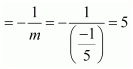Now, the equation of the line passing through point (–3, 5), whose slope is 5, is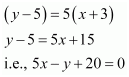Todays Deals### Chapter 10 Straight lines Ex-10.2 Contributorskrishan

Name:
Email:

# Latest News# 9000 interview questions in different categories